# 如何实现高速卷积？深度学习库使用了这些「黑魔法」

2019/12/09 18:22

• 论文地址：https://www.cs.utexas.edu/~flame/pubs/GotoTOMS_revision.pdf

'''Convolve input with kernel to generate output    input.shape = [input_channels, input_height, input_width]    kernel.shape = [num_filters, input_channels, kernel_height, kernel_width]    output.shape = [num_filters, output_height, output_width]'''for filter in 0..num_filters        for channel in 0..input_channels                for out_h in 0..output_height                        for out_w in 0..output_width                                for k_h in 0..kernel_height                    *for* k_w *in* 0..kernel_width                        output[filter, channel, out_h, out_h] +=                             kernel[filter, channel, k_h, k_w] *                             input[channel, out_h + k_h, out_w + k_w]

FLOP/s

• 2个物理内核；

• 每个内核的频率为2.5 GHz，即每秒运行2.5×10^9个CPU循环；

• 每个循环可处理32 FLOPs（使用AVX & FMA）。GFLOP/s。这就是我的CPU的理论峰值性能。类似地，我们可以得出单核CPU的峰值性能为80 GFLOP/s。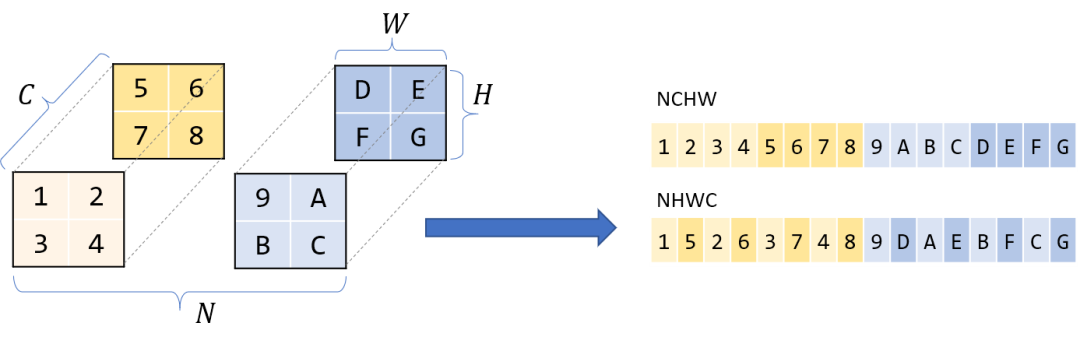Halide语言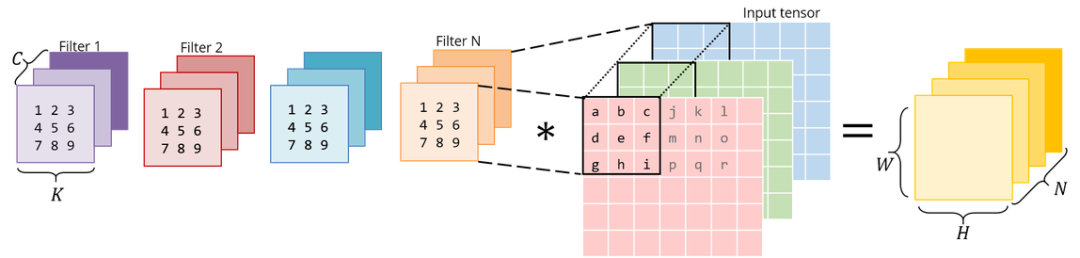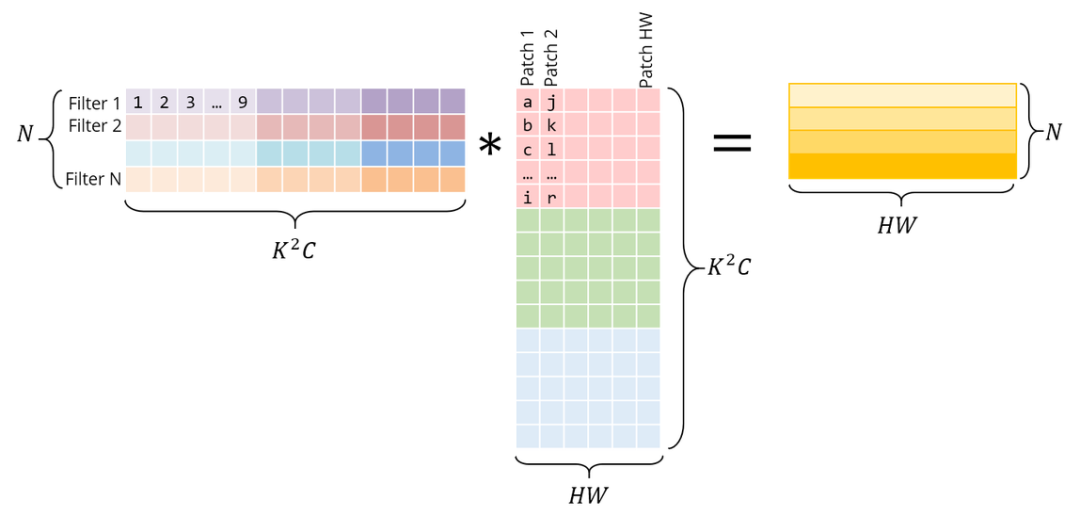for i in 0..M:        for j in 0..N:                for k in 0..K:                        C[i, j] += A[i, k] * B[k, j]

Halide::Buffer C, A, B;Halide::Var x, y;C(x,y) += A(k, x) *= B(y, k);  // loop bounds, dims, etc. are taken care of automatically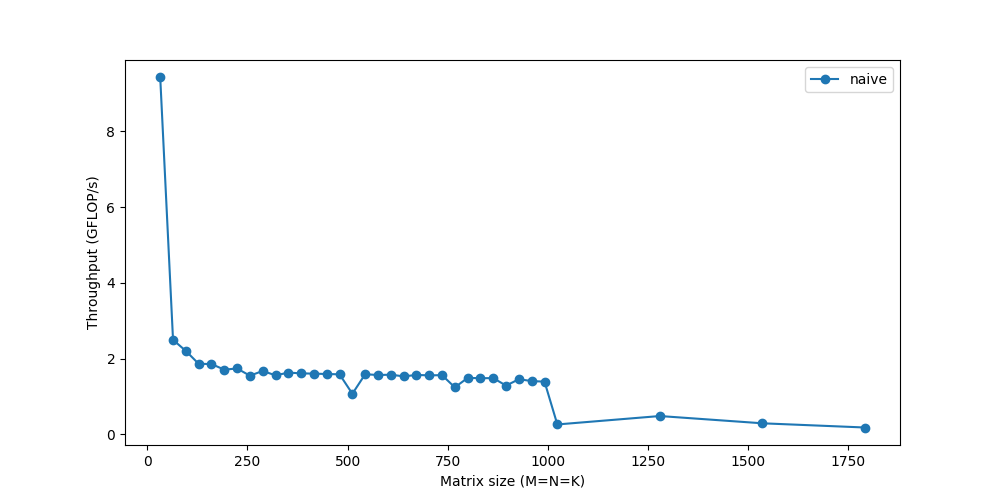RAM是大的存储空间，但速度较慢。CPU缓存的速度要快得多，但其规模较小，因此恰当地使用CPU缓存至关重要。但是并不存在明确的指令：「将该数据加载到缓存」。该过程是由CPU自动管理的。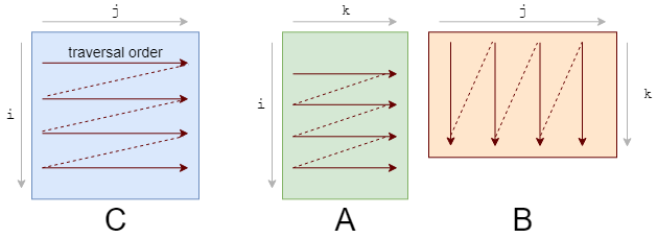• 列的下一个元素并未出现在缓存中，即出现了缓存缺失（cache miss）。这时尽管获取到了数据，CPU也出现了一次停顿。

• 获取数据后，缓存同时也被 B 中同一行的其他元素填满。我们实际上并不会使用到它们，因此它们很快就会被删除。多次迭代后，当我们需要那些元素时，我们将再次获取它们。我们在用实际上不需要的值污染缓存。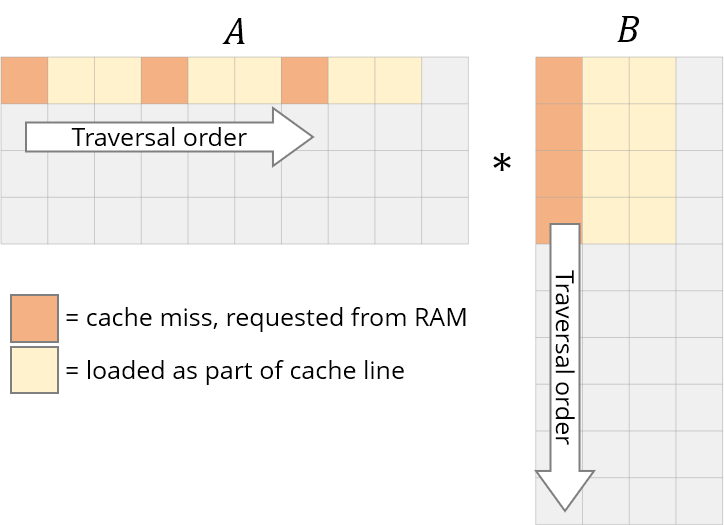for i in 0..M:        for k in 0..K:                for j in 0..N: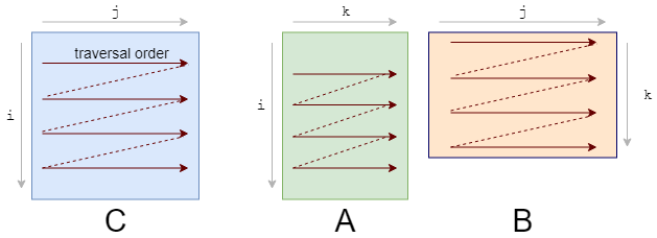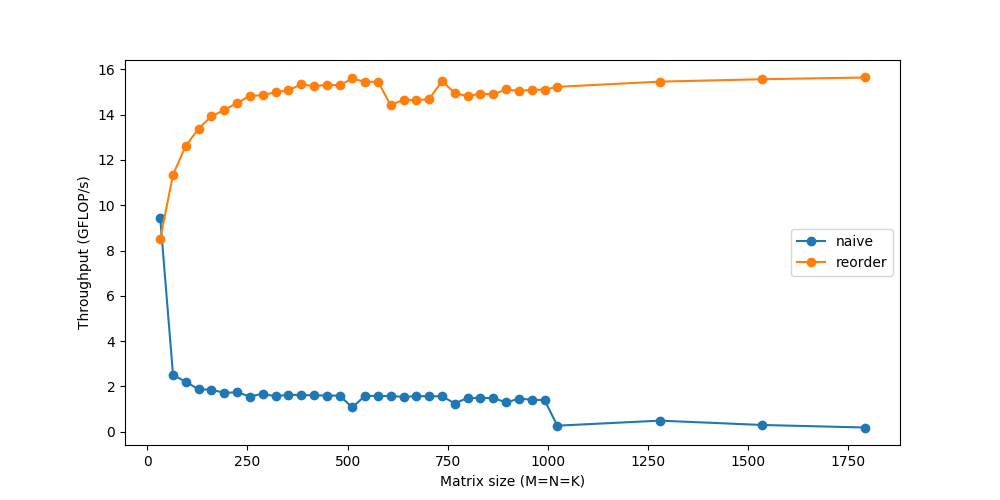C(x, y) += A(k, y) * B(x, k);C.update().tile(x, y, xo, yo, xi, yi, 6, 16)/*in pseudocode:for xo in 0..N/16:    for yo in 0..M/6:            for yi in 6:                    for xi in 0..16:                            for k in 0..K:                                    C(...) = ...*/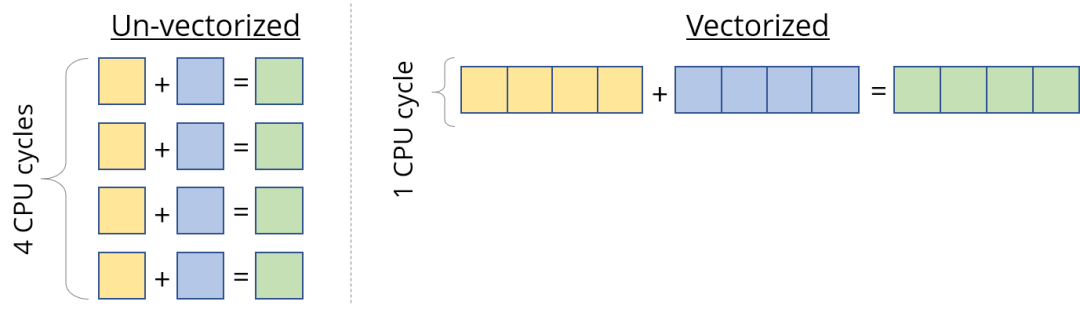C.update().tile(x, y, xo, yo, xi, yi, 6, 16).reorder(xi, yi, k, xo, yo).vectorize(xi, 8)/*in pseudocode:for xo in 0..N/16:    for yo in 0..M/6:            for k in 0..K:                    for yi in 6:                            for vectorized xi in 0..16:                                    C(...) = ...*/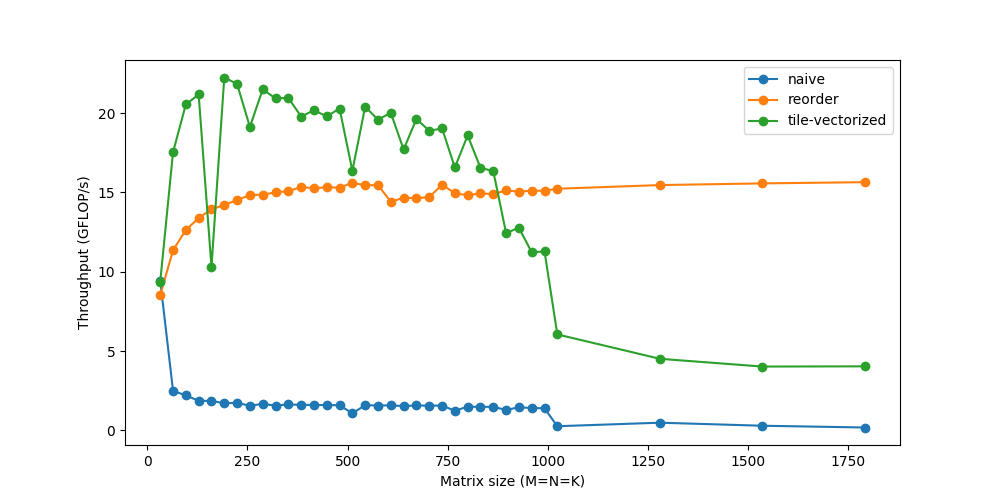C.update().tile(x, y, xo, yo, xi, yi, 6, 16).reorder(xi, yi, k, xo, yo).vectorize(xi, 8).parallel(yo)/*in pseudocode:for xo in 0..N/16 in steps of 16:    for parallel yo in steps of 6:            for k in 0..K:                    for yi in 6:                            for vectorized xi in 0..16 in steps of 8:                                    C(...) = ...*/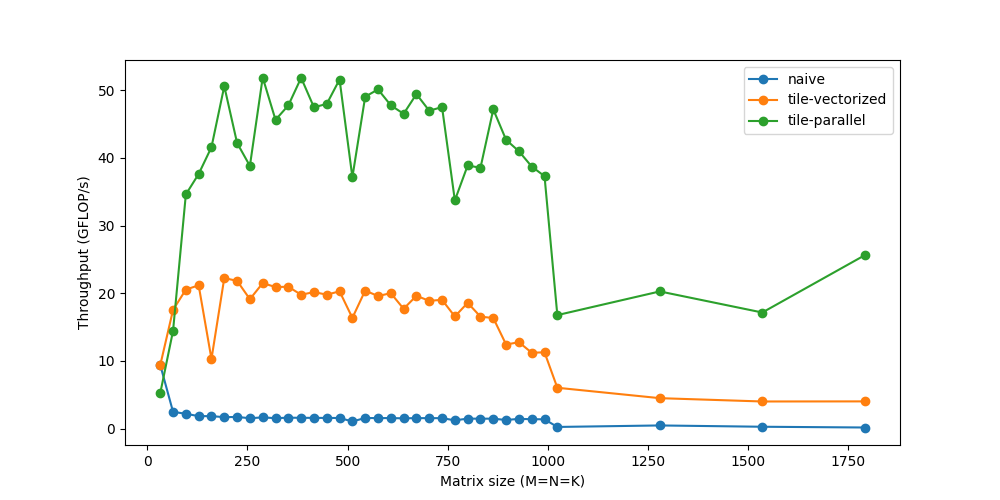C.update().tile(x, y, xo, yo, xi, yi, 6, 16).reorder(xi, yi, k, xo, yo).vectorize(xi, 8).unroll(xi).unroll(yi)/*in pseudocode:for xo in 0..N/16:    for parallel yo:            for k in 0..K:                    C(xi:xi+8, yi+0)                    C(xi:xi+8, yi+1)                    ...                    C(xi:xi+8, yi+5)                    C(xi+8:xi+16, yi+0)                    C(xi+8:xi+16, yi+1)                    ...                    C(xi+8:xi+16, yi+5)*/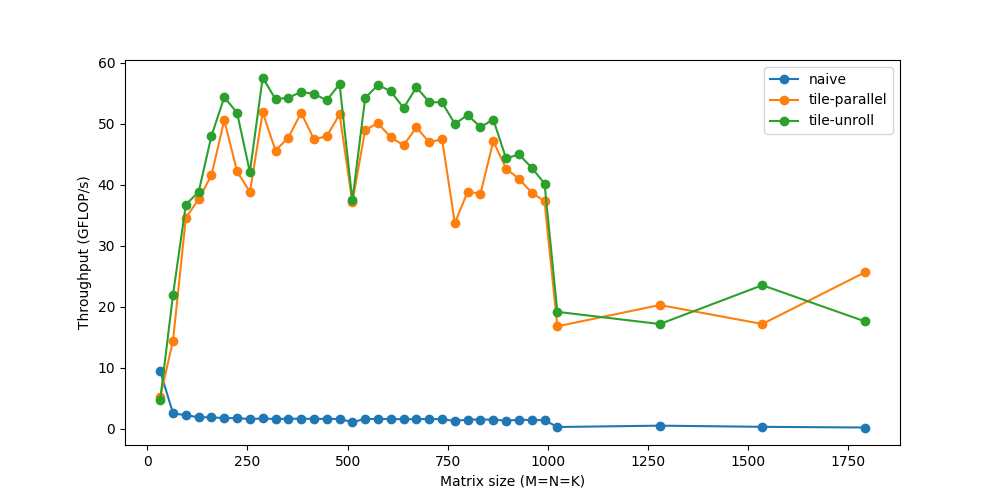matrix_mul(x, y) += A(k, y) * B(x, k);    out(x, y) = matrix_mul(x, y);out.tile(x, y, xi, yi, 24, 32)          .fuse(x, y, xy).parallel(xy)          .split(yi, yi, yii, 4)          .vectorize(xi, 8)          .unroll(xi)          .unroll(yii); matrix_mul.compute_at(out, yi)           .vectorize(x, 8).unroll(y); matrix_mul.update(0)           .reorder(x, y, k)           .vectorize(x, 8)           .unroll(x)           .unroll(y)           .unroll(k, 2);

1. 将out分解为32x24的平铺，然后将每个平铺进一步分解为8x24的子平铺。

2. 使用类似的重排序、向量化和展开，在临时缓冲区（matrix_mul）计算8x24 matmul。

3. 使用向量化、展开等方法将临时缓冲区matrix_mul 复制回out。

4. 在全部32x24平铺上并行化这一过程。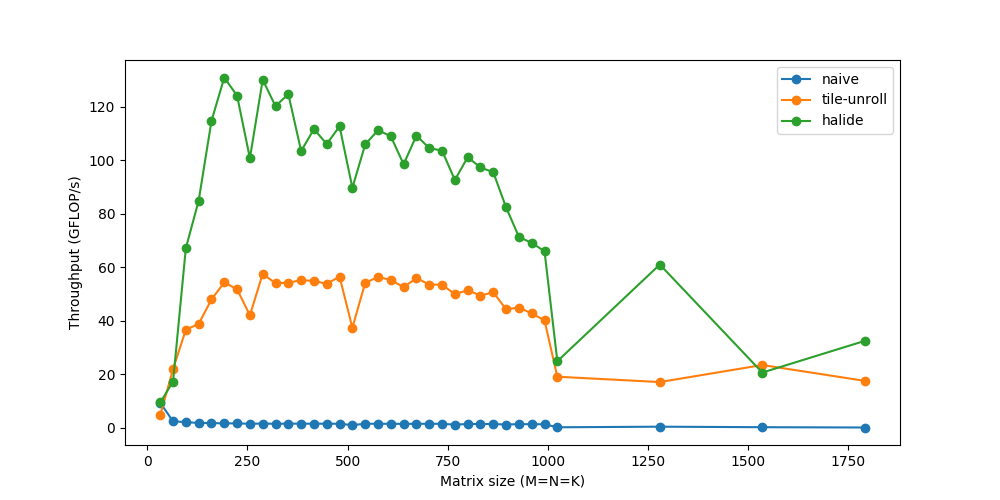https://sahnimanas.github.io/post/anatomy-of-a-high-performance-convolution/

0
0 收藏

### 作者的其它热门文章0 评论
0 收藏
0